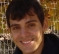Started by 6 years ago8 replieslatest reply 6 years ago250 views

Can a Quadrature Modulator be used to generate ASK (Amplitude Shift Keying) ?

More generally, is there a hardware to generate both BPSK and ASK?

[ - ]What are you trying to do, and with what knowledge base?

There are quadrature up-converter chips which can be used to generate ASK, yes.  That's such an obvious "yes" though that I wonder what you're thinking of when you say "quadrature modulator".

And there is all sorts of hardware that'll generate BPSK and ASK, ranging from a processor (DSP or FPGA) connected to an ADC or two, all the way down to a small board with TTL logic and resistors.

So -- what are you trying to do, and with what knowledge base?

[ - ]I can understand how Quadrature Modulator (like ADRF6755) can be used to generate BPSK, QPSK, MSK etc by controlling the I and Q signals that feed it. But how will it generate FSK and ASK. Can you explain in detail or give a reference.
[ - ]Well, MSK is FSK, so if you do know how to generate MSK but don't know how to generate FSK then I think maybe you only know how to look in a book that tells you how to generate MSK, but you don't actually understand the process.  Rote learning is not understanding.

Calculate $$e^{j \omega(t) t}$$.  Apply the real part to the inphase input.  Apply the imaginary part to the quadrature input.  The output is your carrier, frequency shifted by $$\omega(t)$$.  Now you have what you need to understand MSK and FSK.

For ASK, just apply a unipolar signal to either the I or Q input -- you'll amplitude modulate the carrier.

In theory, if it can be transmitted over the air, you can generate anything with one of those chips.  There are practical limitations having to do with how well the I and Q channels are matched in phase and amplitude, but remember -- anything.

[ - ]MSK is CPFSK. It can also be seen as a form of QPSK (with pulse shaping of OQPSK). If you go by QPSK interpretation, it can implemented by a circuit which otherwise can not generate FSK. Thats why i asked the question.

Secondly i have not found any reference for generating FSK as explained by you using a commercial Quadrature Modulator chip. Can you share if you have any?

[ - ]The details of doing this all came out as I was working my career, so I've just kind of absorbed it.  If it's not in a book on designing radios, then it should be in various applications notes.

Try searching on "the phasing method" and "the Weaver method".  You'll get a lot of hits on amateur single-sideband radio design, but those hits will explain the concept, too.

The reason that it works is because the quadrature modulator acts as if it is performing the following function:

$x(t) = \mathcal{R}\left\{ A(t) e^{j 2\pi f t} \right\}$

where the real and imaginary parts of $$A(t)$$ are what you plug into the inphase and quadrature parts of the modulator.  Everything else follows from that.

[ - ]Yes, a quadrature modulator can be used to generate all kind of modulation, as long as you can control the I and Q signals that feed it. It allows you to convert the I and Q base band signal directly to the target transmit frequency.

As Tim Wescott said, you may have a FPGA, DSP or Microcontroller controlling two ADCs, one for the I signal and other for the Q signal. Them, you may do any kind of modulation scheme.

Now, if you are interested only in BPSK and ASK signal, you can use something more simple than a quadrature modulator to make a direct conversion.

[ - ]